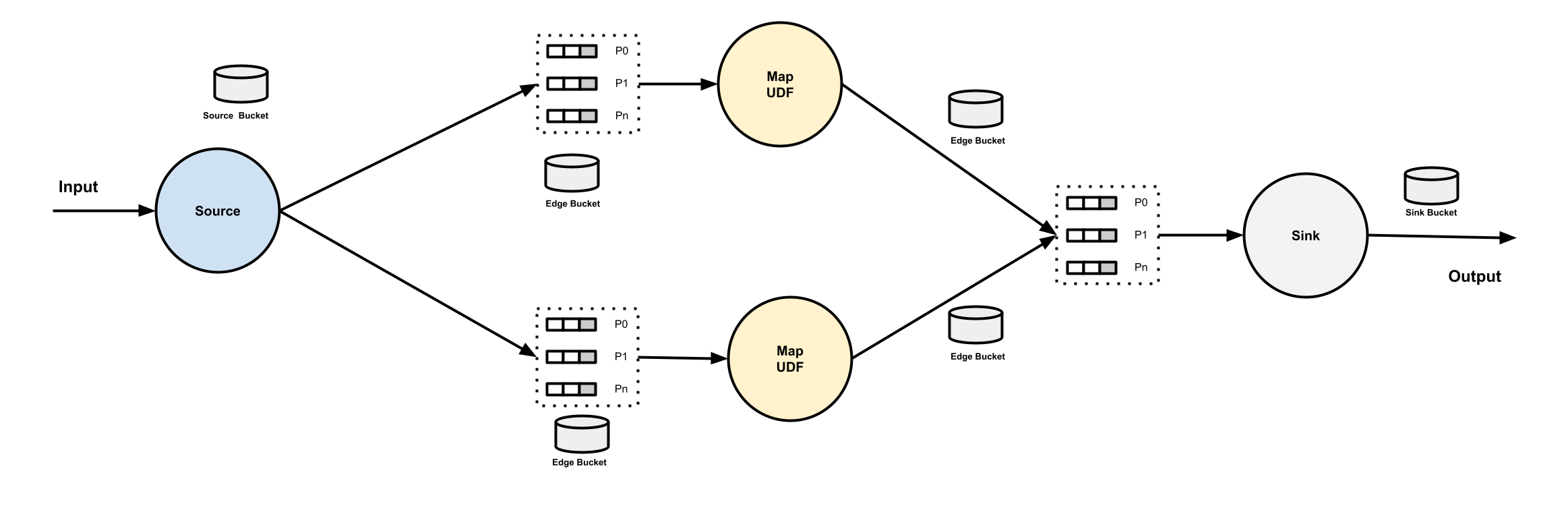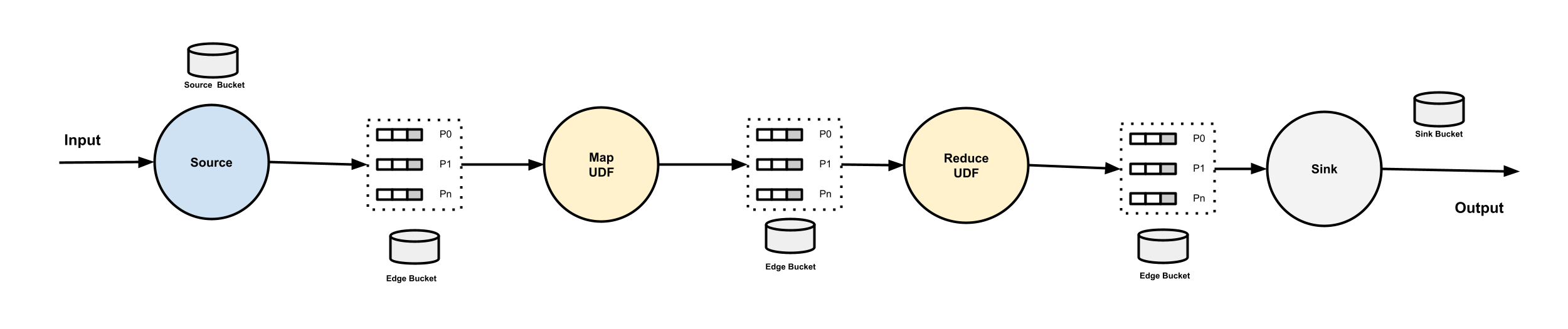# Edges, Buffers and Buckets¶This document describes the concepts of `Edge`, `Buffer` and `Bucket` in a pipeline.

## Edges¶

`Edge` is the connection between the vertices, specifically, `edge` is defined in the pipeline spec under `.spec.edges`. No matter if the `to` vertex is a Map, or a Reduce with multiple partitions, it is considered as one edge.

In the following pipeline , there are 3 edges defined (`in` - `aoti`, `aoti` - `compute-sum`, `compute-sum` - `out`).

``````apiVersion: numaflow.numaproj.io/v1alpha1
kind: Pipeline
name: even-odd-sum
spec:
vertices:
- name: in
source:
http: {}
- name: atoi
scale:
min: 1
udf:
container:
image: quay.io/numaio/numaflow-go/map-even-odd
- name: compute-sum
udf:
container:
image: quay.io/numaio/numaflow-go/reduce-sum
groupBy:
window:
fixed:
length: 60s
keyed: true
- name: out
scale:
min: 1
sink:
log: {}
edges:
- from: in
to: atoi
- from: atoi
to: compute-sum
parallelism: 2
- from: compute-sum
to: out
``````

Each `edge` could have a name for internal usage, the naming convention is `{pipeline-name}-{from-vertex-name}-{to-vertex-name}`.

## Buffers¶

`Buffer` is `InterStepBuffer`. Each buffer has an owner, which is the vertex who reads from it. Each `udf` and `sink` vertex in a pipeline owns a group of partitioned buffers. Each buffer has a name with the naming convention `{pipeline-name}-{vertex-name}-{index}`, where the `index` is the partition index, starting from 0. This naming convention applies to the buffers of both map and reduce udf vertices.

When multiple vertices connecting to the same vertex, if the `to` vertex is a Map, the data from all the from vertices will be forwarded to the group of partitoned buffers round-robinly. If the `to` vertex is a Reduce, the data from all the from vertices will be forwarded to the group of partitoned buffers based on the partitioning key.

A Source vertex does not have any owned buffers. But a pipeline may have multiple Source vertices, followed by one vertex. Same as above, if the following vertex is a map, the data from all the Source vertices will be forwarded to the group of partitoned buffers round-robinly. If it is a reduce, the data from all the Source vertices will be forwarded to the group of partitoned buffers based on the partitioning key.

## Buckets¶

`Bucket` is a K/V store (or a pair of stores) used for watermark propagation.

There are 3 types of buckets in a pipeline:

• `Edge Bucket`: Each edge has a bucket, used for edge watermark propagation, no matter if the vertex that the edge leads to is a Map or a Reduce. The naming convention of an edge bucket is `{pipeline-name}-{from-vertex-name}-{to-vertex-name}`.
• `Source Bucket`: Each Source vertex has a source bucket, used for source watermark propagation. The naming convention of a source bucket is `{pipeline-name}-{vertex-name}-SOURCE`.
• `Sink Bucket`: Sitting on the right side of a Sink vertex, used for sink watermark. The naming convention of a sink bucket is `{pipeline-name}-{vertex-name}-SINK`.

## Diagrams¶

MapReduce